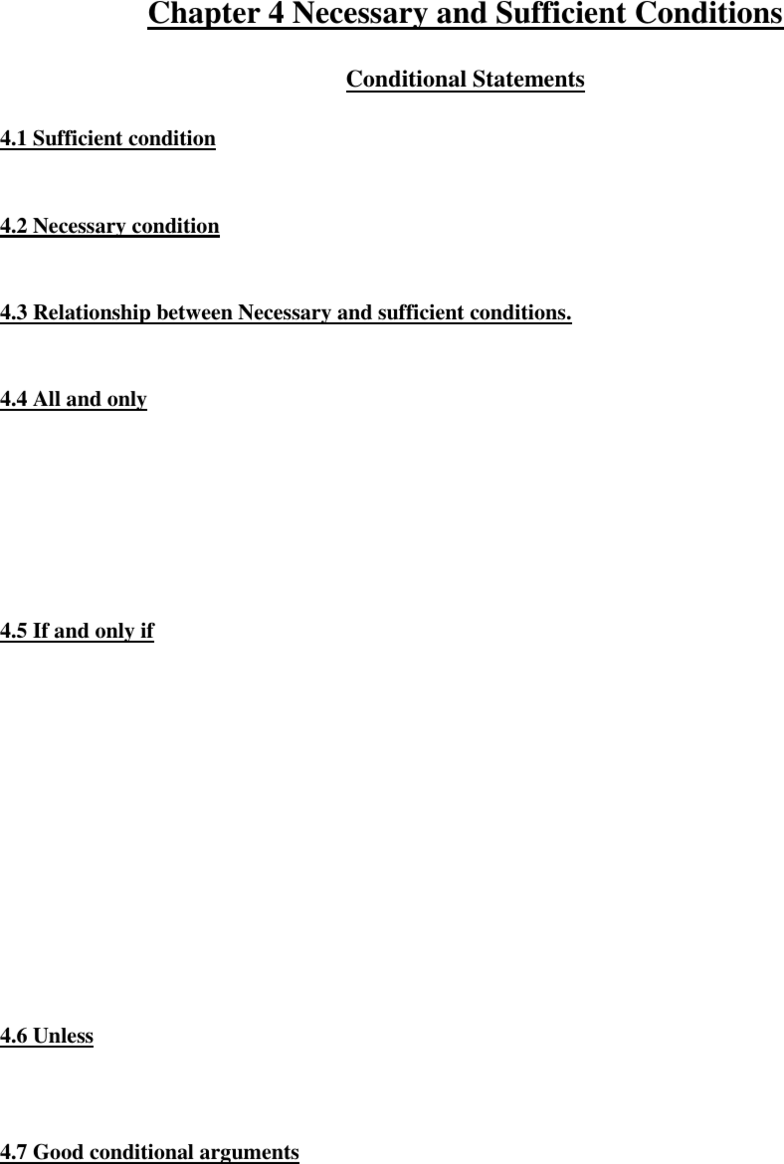Textbook Notes (280,000)
CA (160,000)
Ryerson (10,000)
PHL (200)
PHL 214 (70)
Chapter 4

# Chapter 4 Necessary and Sufficient Conditions.docx

Department
Philosophy
Course Code
PHL 214
Professor
James Cunningham
Chapter
4

Page:
of 2Chapter 4 Necessary and Sufficient Conditions
Conditional Statements
4.1 Sufficient condition
- A is sufficient for B if A is true, then B is true.
4.2 Necessary condition
- X is necessary condition for Y: if X is not true, Y is not true.
4.3 Relationship between Necessary and sufficient conditions.
- A is sufficient for B = B is necessary for A.
4.4 All and only
- All A’s are B’s
= A is sufficient for B
= B is necessary for A
- Only X’s are Y’s
= X is necessary for Y
= Y is sufficient for X
4.5 If and only if
- Antecedent comes immediately after the, if.
- Consequent is the necessary condition.
- ALL OF THE FOLLOWING MEAN THE SAME THING:
If S, then N
N, if S
S only if N
Only S if N
- If you are a student, then you’re a human.
- You’re human if you’re a student.
- You’re a student only if you’re a human.
- Being a student is sufficient for being human.
- Being human is necessary for being a student.
4.6 Unless
- Not A unless B =B is necessary for A.
- A unless B = B is necessary for not A.
4.7 Good conditional arguments
- Affirm the sufficient condition (Modus ponens)
- Deny the necessary condition (Modus tollens)
- “Affirm” means “say the same thing”
- “Deny” means “say the opposite”